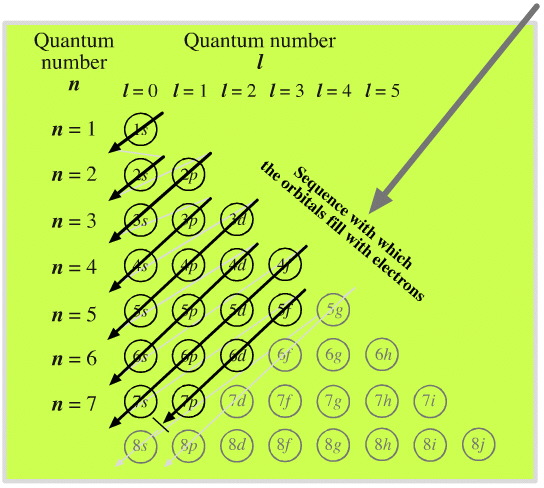# What is the Madelung's rule?

May 2, 2015

1. The energy of an atomic orbital increases with increasing $n + l$
2. For identical values of $n + l$, energy increases with increasing $n$

where $n$ is the principal quantum number and $l$ is the azimuthal quantum number.

Identical values of $n + l$

For a $2 p$ orbital, $n + l = 3$; for a $3 s$ orbital, $n + l = 3$. So $2 p < 3 s$.

If there are identical $n + l$ values, the orbital with a lower $n$ value is filled first.

Different $n + l$ values

For a $3 d$ orbital, $n + l = 5$; for a $4 s$ orbital, $n + l = 4$, so $4 s < 3 d$.

If there are different $n + l$ values, the orbital with a lower $n + l$ value is filled first.

This order is based on the total number of nodes in the atomic orbital, $n + l$.

The higher the value of $l$, the more nodes, and the higher the energy of the orbital.

The complete order for filling orbitals according to the Madelung rule is:

$1 s$, $2 s 2 p$, $3 s 3 p$, $4 s 3 d 4 p$, $5 s 4 d 5 p$, $6 s 4 f 5 d 6 p$, $7 s 5 f 6 d 7 p$, $8 s 5 g 6 f 7 d 8 p$, 9s…

The order is summarized in the triangular diagram below.